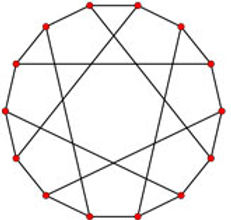top of page### A graph is a mathematical abstraction of a network.

Graphs are used to describe complex system in almost every area of business, industry and technology. Similarly, a group is the mathematical abstraction of the symmetry of an object, and groups are used in many branches of mathematics, as well as in physics, chemistry and networks.

Take for example these graphs:Heawood graphPetersen graph

Algebraic Graph Theory: TeamMember

There are various ways you could rotate these graphs and keep them looking the same. This is what we mean when we say that a graph is symmetric. This graph comes equipped with a group; the algebraic object which measures the symmetry of the graph.

Studying the group action of a graph is an important method for studying both the group and the graph. That is, we investigate the group through its action on the graph, and we study the graph by the properties of its group. This forms an important mathematical method and has produced various important mathematics results.

For example, it led to the proof of Weiss' Theorem that there exists no 8-arc-transitive graph, and the proof of Cameron-Praeger-Seitz-Saxl's theorem which tells us that there are only finitely many distance-transitive graphs of a given valency.

On the other hand, Goldschmidt's study of groups acting on cubic graphs led to the creation of the amalgam method (an important method for characterising certain finite simple groups), and Fong and Seitz's study of Moufang polygons (a special type of graph) led to the characterisation of rank-2 Lie type groups.

## RESEARCH

Our Centre is a world leading centre in algebraic graph theory, with world experts. The research work at the Centre covers important topics in algebraic graph theory, such as:

• Cayley graphs

• distance-transitive graphs

• s-arc-transitive graphs

• symmetric graphs

• half-arc-transitive graphs

• symmetrical factorisations of graphs

• symmetrical embeddings of graphs on Riemann surfaces.

Members of the Centre have made significant contributions to the area. They have solved various important long-standing open problems, established significant theories, developed powerful methods and launched new research directions, including:

• Praeger's theory of 2-arc-transitive graphs, and later developed Giudic-Li-Praeger theory of locally-s-arc-transitive graphs, provide major methods for analysing edge-transitive graphs.

• The Li-Praeger theory of homogeneous factorisations of graphs launched a new research direction in combinatorics.